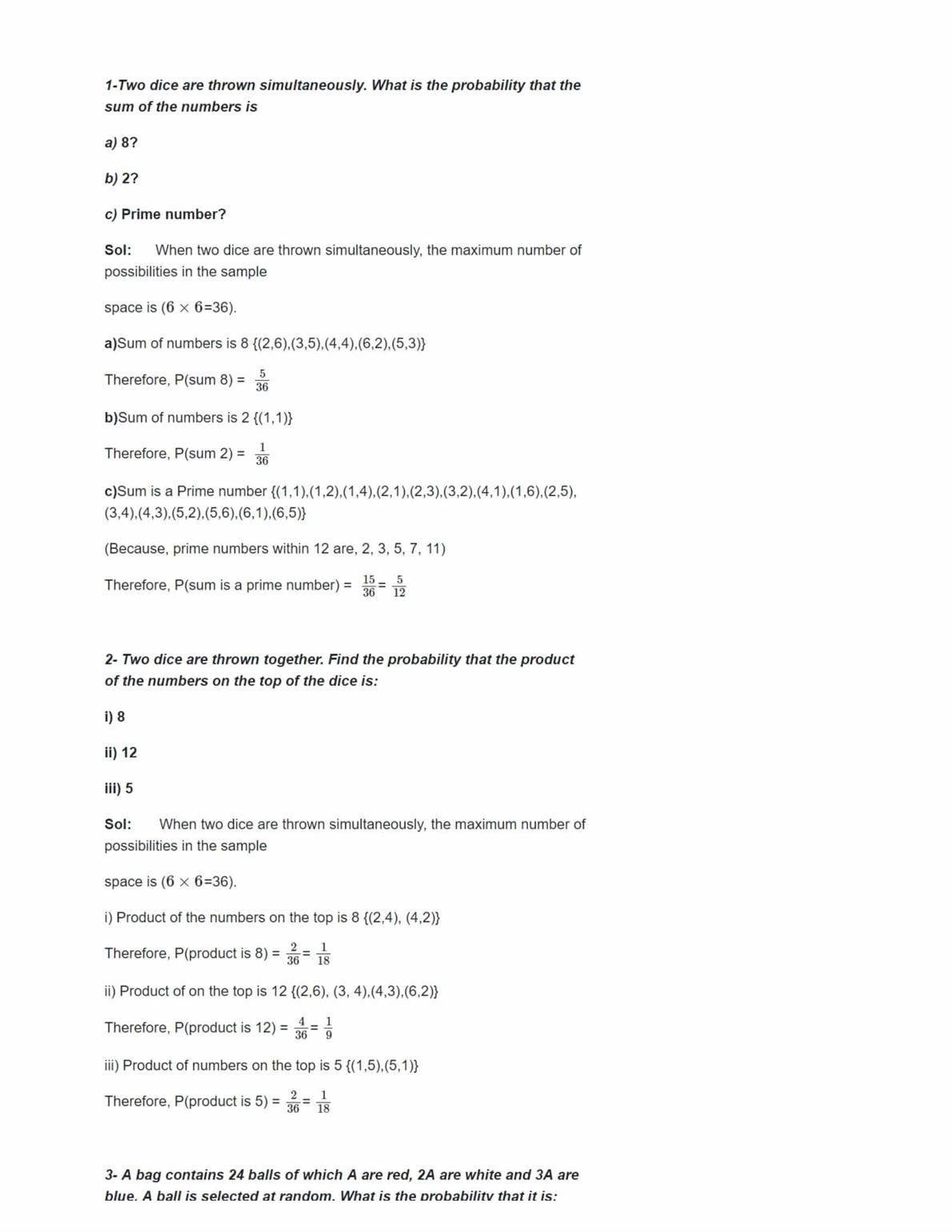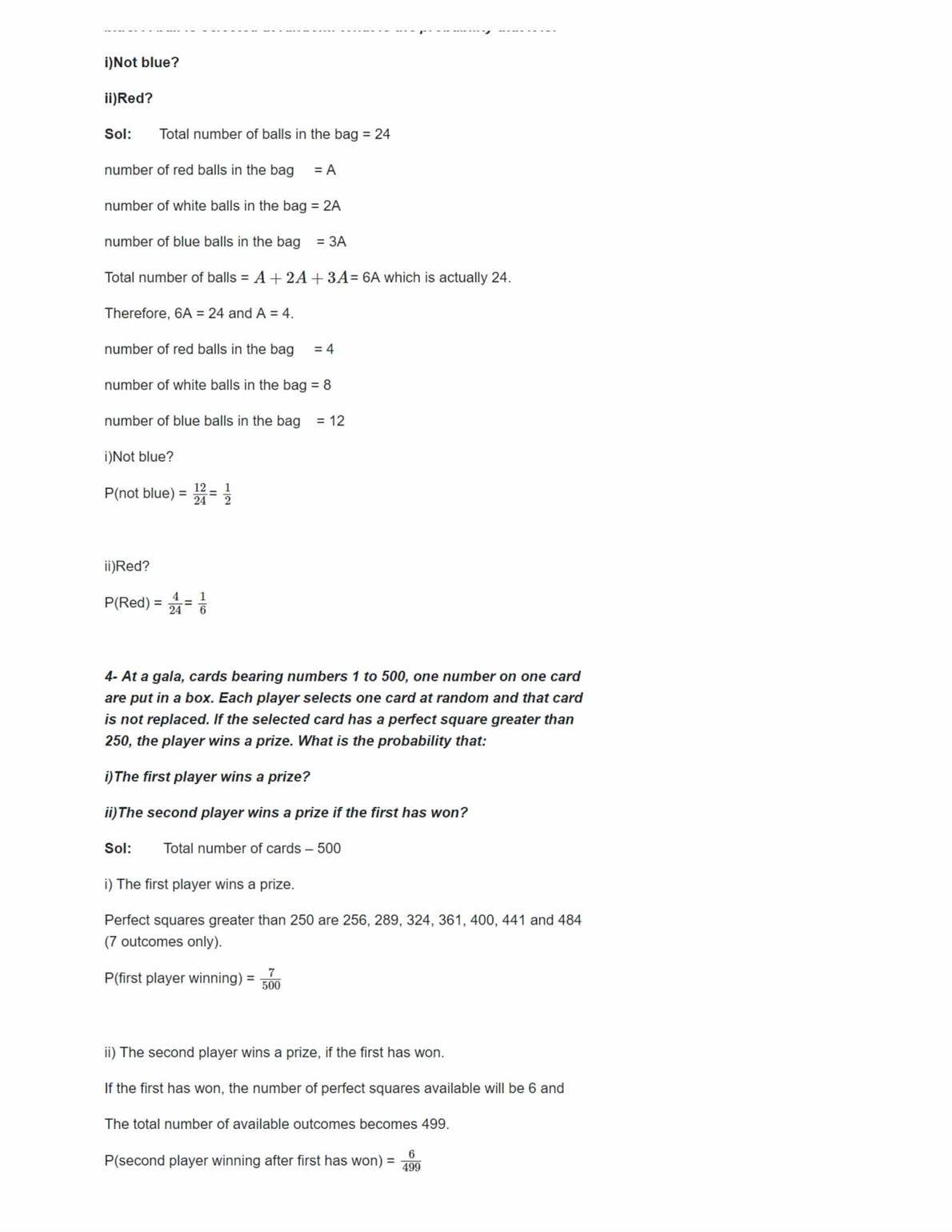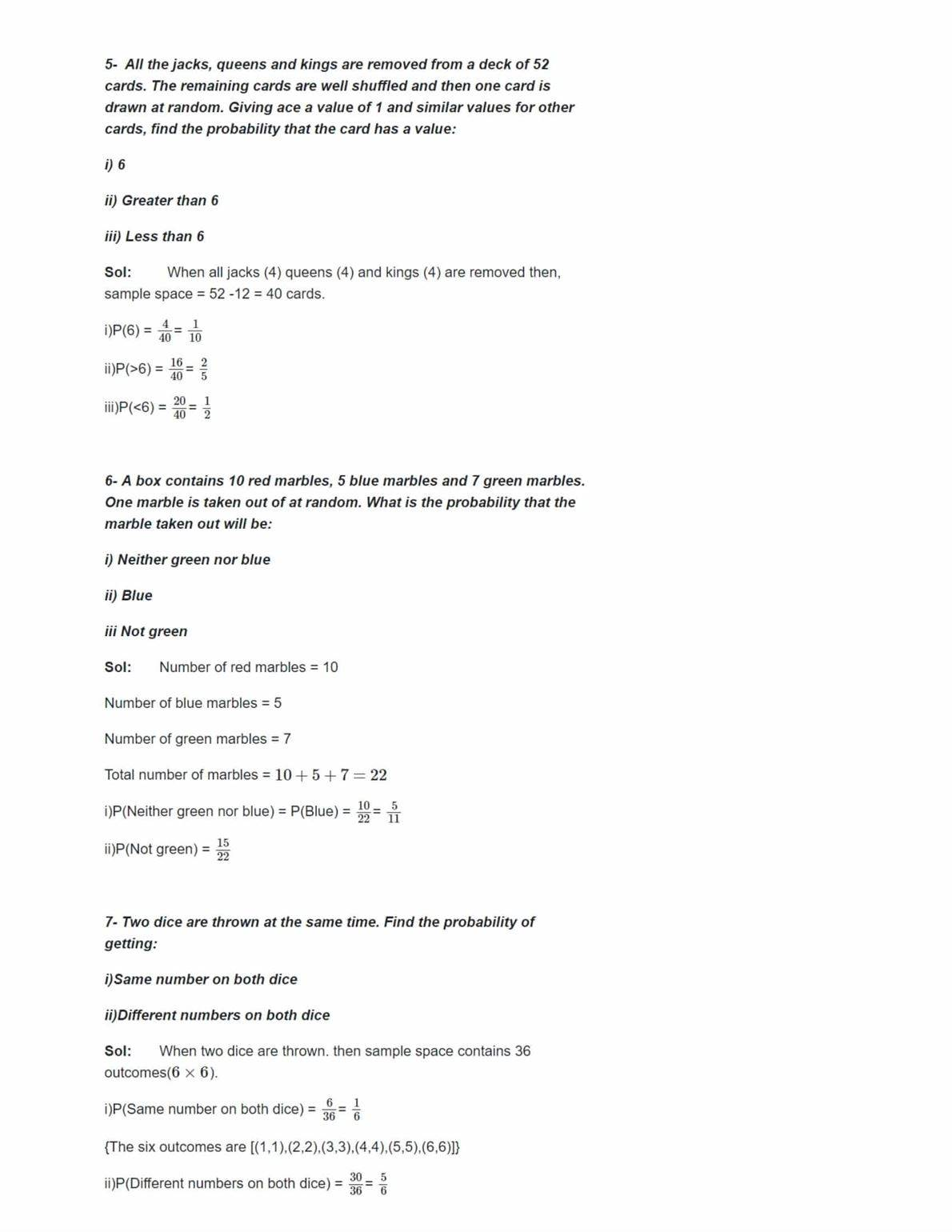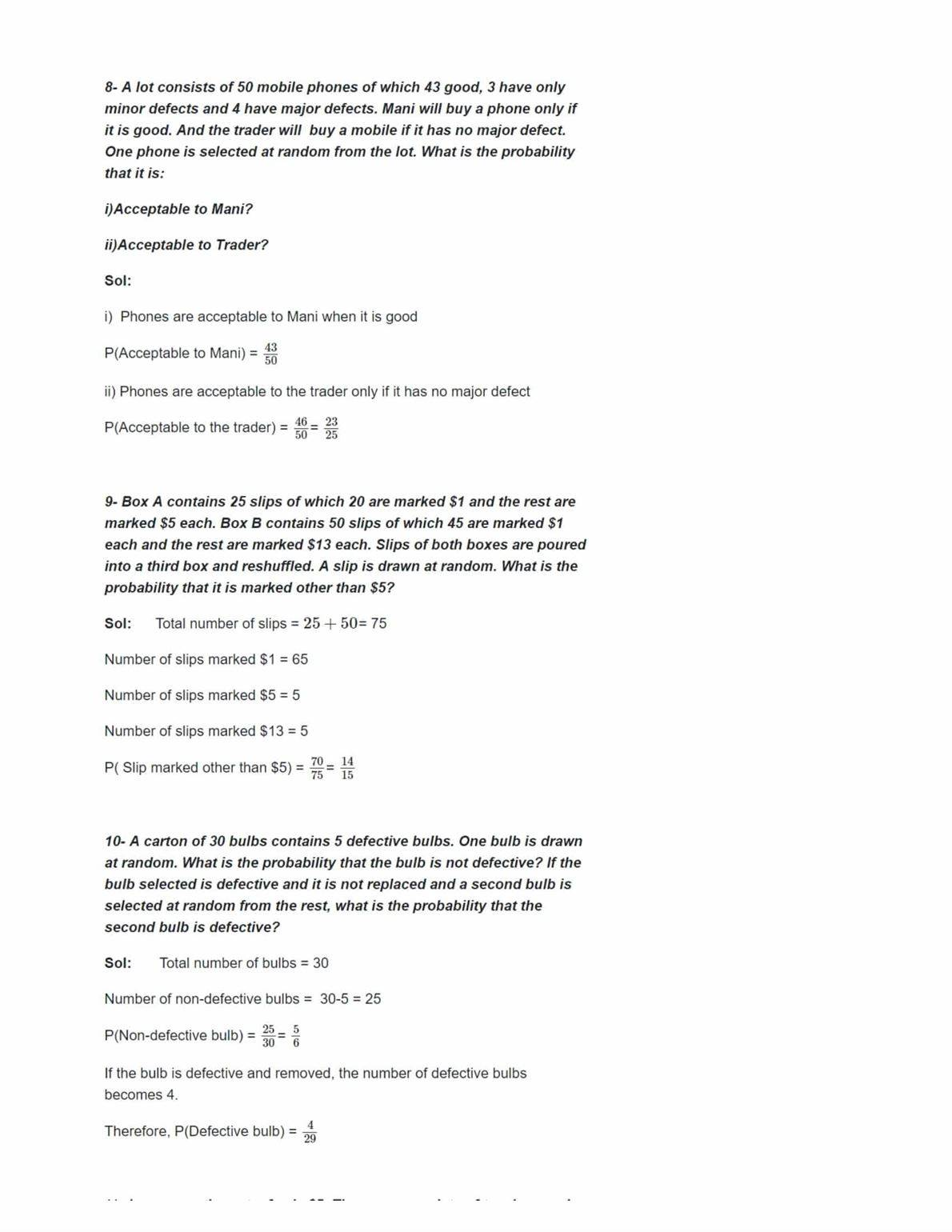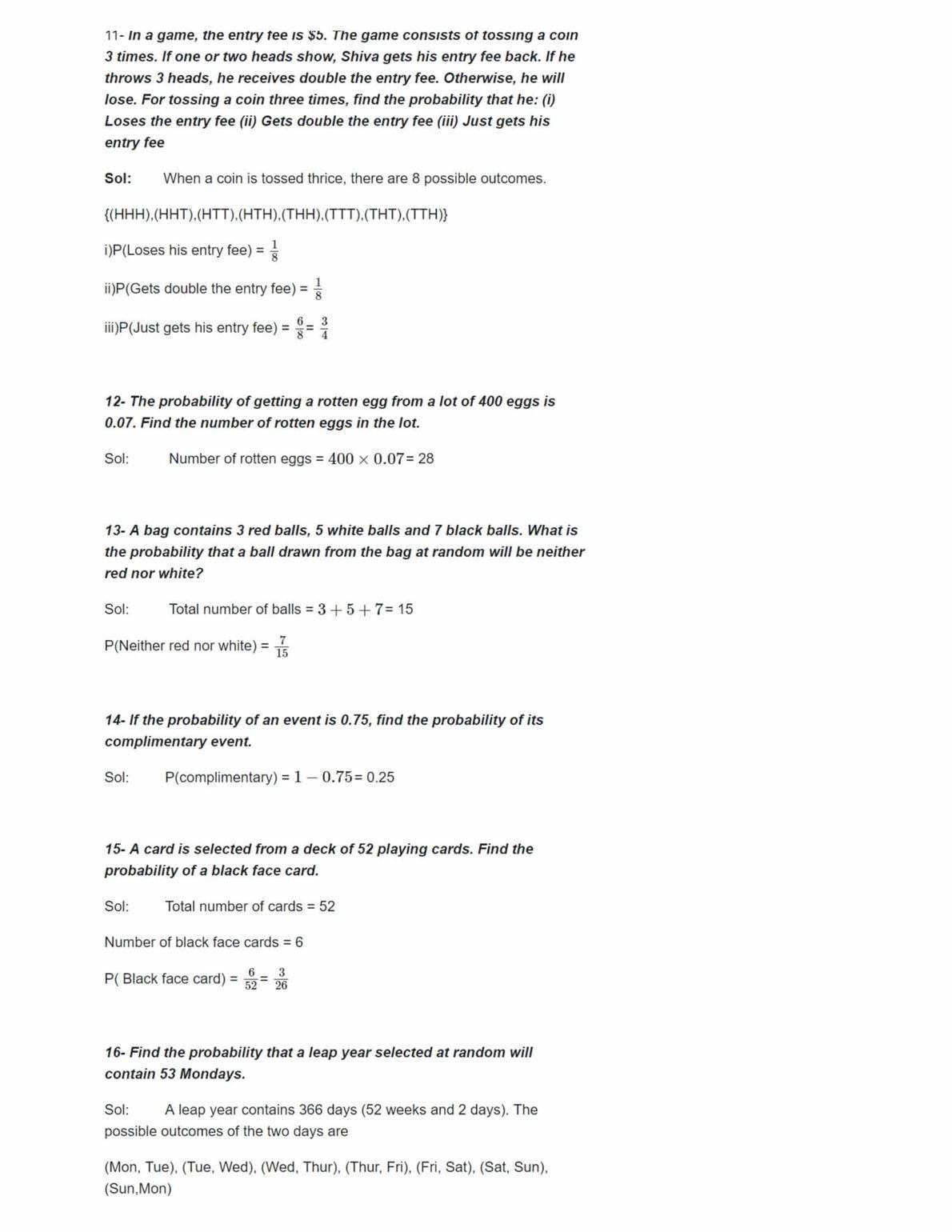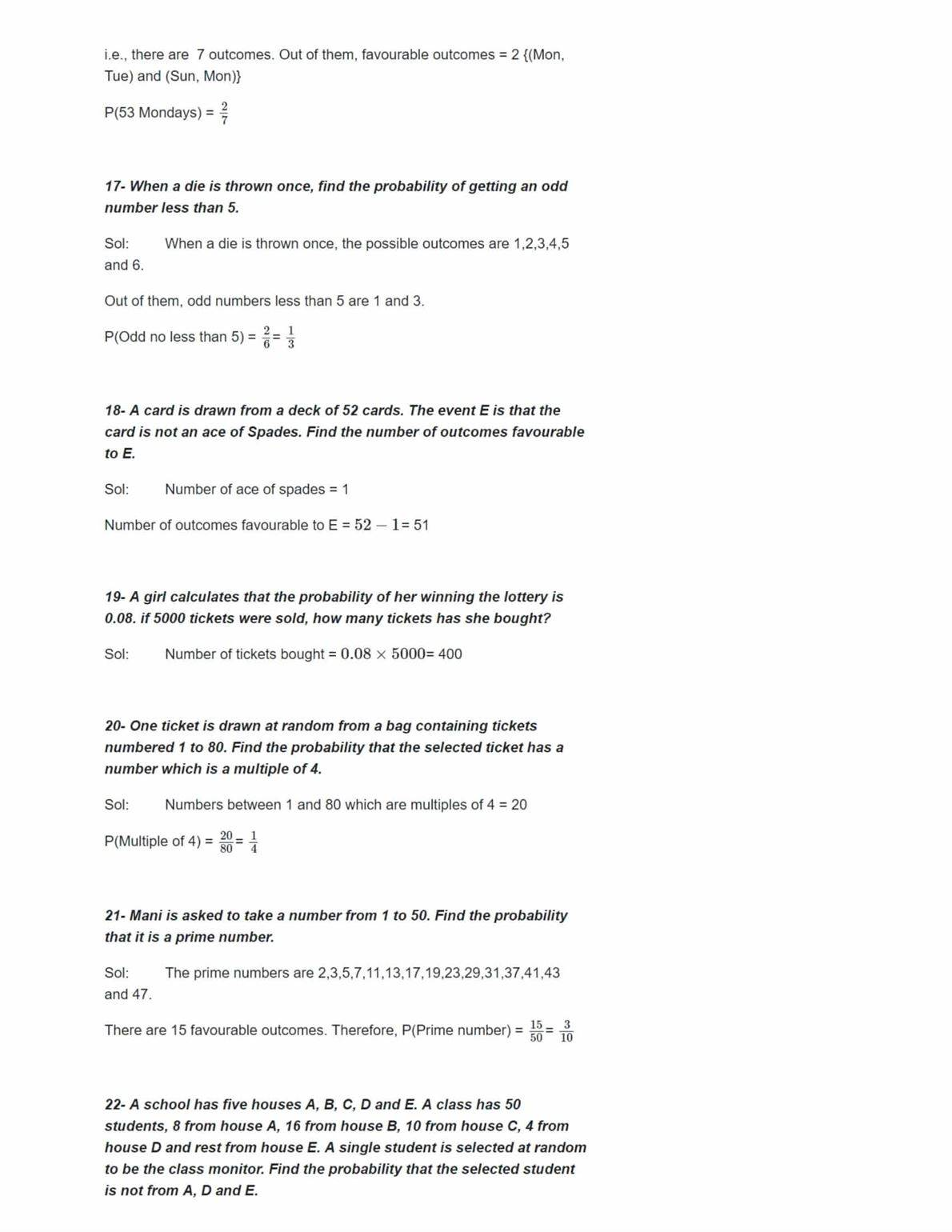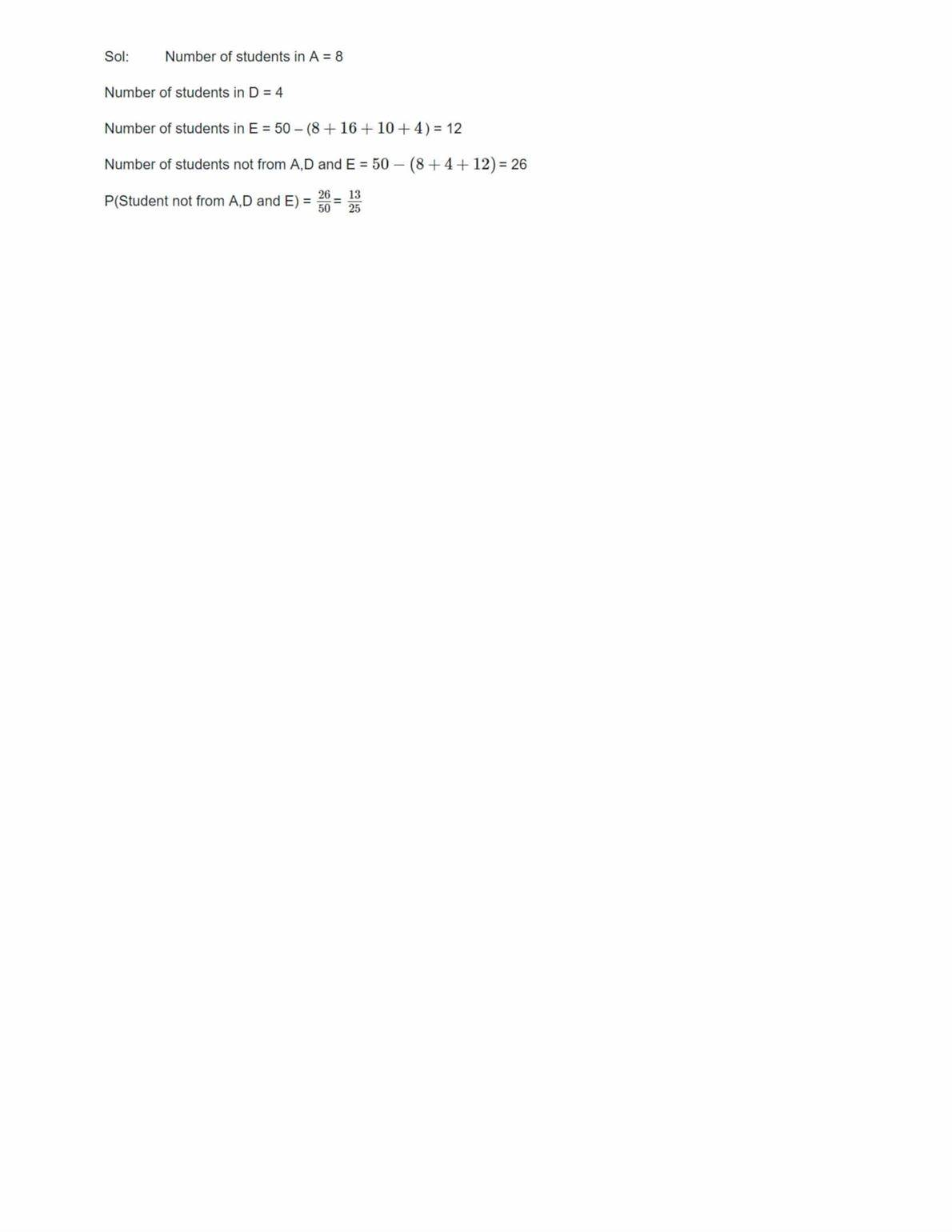# NCERT Solutions Class 10 Maths Chapter 15 – Download PDF

Get here NCERT Solutions Class 10 Maths Chapter 15. These NCERT Solutions for Class 10 of Maths subject includes detailed answers of all the questions in Chapter 15 – Probability provided in NCERT Book which is prescribed for class 10 in schools.

Resource: National Council of Educational Research and Training (NCERT) Solutions
Class: 10th Class
Subject: Maths
Chapter: Chapter 15 – Probability

## NCERT Solutions Class 10 Maths Chapter 15- Probability

The NCERT textbooks are undoubtedly an essential study material for the students studying in CBSE Class 10. NCERT solutions of Class 10 Chapter 15 of maths, Probability, is provided here along with the downloadable PDF. The chapter is a continuation of what was taught in chapter probability in Class 9 and further explains the different concepts related to it.

The subject experts prepared the NCERT solutions for Class 10 Maths to assist students in preparing for their board examination. Every step and concept used in solving a solution is explained clearly in the answers provided at EXAMIN, leaving no question unsolved. Along with exam preparation, these solutions can be used to check if the answers given to the exercise questions by the students are correct while doing their homework and assignments. So, it is advised to all the students to go through the these NCERT Solutions regularly to stand out among the other students in the class and also to excel in the Board examination of Class 10 standard exam.

Chapter 15 of Class 10, Probability, belongs to the 6th Unit- Statistics and Probability, that adds up to 10 marks out of the total 80 marks in the examination. Unit 6 is one such unit, in which, marks can be easily scored once the method or the idea gets familiar. Solving the NCERT exercise questions will enable the students in getting thorough with the methods used in solving the problems of this chapter, in turn aiding the students to prepare well for the Class 10 Maths Board examination.

Main topics covered in this chapter include:

15.1 Introduction

15.2 Probability – A Theoretical Approach

Exercise 15.1 Solutions 25 Questions (1 MCQ, 21 Short Answer Questions, 2 Long Answer Question, 1 Main Question with 4 Sub-questions)

Exercise 15.2 Solutions 5 Questions (5 Short Answer Questions)

Solving the NCERT Solutions for Class 10 is very important from the CBSE board examination point of view. Probability is one of the chapters in Class 10 maths that contain the concepts that are most likely to be used in daily life. The chapter deals with different topics related to the probability that includes:

1. The difference between experimental probability and theoretical probability
2. Why the probability of a sure event (or certain event) is 1.
3. How the probability of an impossible event is 0?
4. The explanation for the statement:

The probability of an event E is a number P(E) such that

0 ≤ P (E) ≤ 1

1. Elementary events.
2. Complementary events.
3. Finding the probability of different events.
• In-depth knowledge of the chapter/problems is provided in easy language.
• Is based on the CBSE syllabus.
• Best study material to prepare for the Class 10 board exam.
• All the doubts that might arise while solving the exercise questions will vanish by going through these solutions.